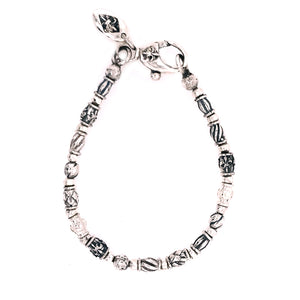Liquid error (image-logic line 10): divided by 0Liquid error (image-logic line 10): divided by 0
Liquid error (image-logic line 10): divided by 0
Liquid error (image-logic line 10): divided by 0
Liquid error (image-logic line 10): divided by 0
Liquid error (image-logic line 10): divided by 0
Liquid error (image-logic line 10): divided by 0

# Silver Bracelet Mini TUBES DECOR and Mini Balls

Elfcraft

Product SKU: 286.00

• €198,00
Unit price per
Tax included.

Carabiner lock and Silver tubes with Elfin´symbols, nice to cobine with 219.000.04Li